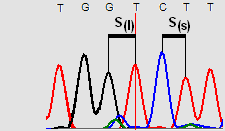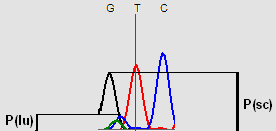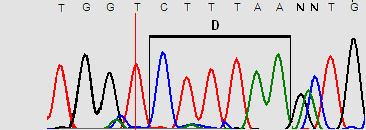Insilicase
deus ex computaChromosome lengthsConsensus site finderDegenerate motif finderExon finderFusion protein (RE)Fusion protein (PCR)Genetic distanceHeterozygous InDelsInvert sequenceList comparisonMicrosatellite peaksMismatch TmMutagenesis (silent)Mutation severityORF annotationPhred score calculationProtein cleavage fragmentsRandom sequenceResidue codes etcRestriction sitesRFLP enzyme pickerStats decision tree

# Phred score calculation

This page is designed to help explain how a PHRED score is derived from four parameters that are calculated as described below:Peak spacingFor each window of 7 bases the largest and smallest spaces between two peaks are found and used to calculate the ratio of the largest space divided by smallest space (S(l)/S(s)). If the sequence is evenly spaced this ratio equals 1. Figure 1Uncalled to called peak height ratio For each window of 7 bases the ratio of the height of the largest uncalled peak (P(lu)) against the height of the shortest called peak (P(sc)) is found. If no uncalled peak is present in the window then the highest background value under a peak is used. Figure 2Uncalled to called peak height ratio in a three base windowThis is calculated the same as the last parameter, but uses the values from a 3 base window. Figure 3Peak resolutionThe number of bases between the current base and the nearest unresolved base (i.e. a base that is called as ‘N’) is found and then multiplied by -1. Figure 4

These parameters were found in a large training set of 10s of thousands of sequences where the correct base was known. By finding how often the correct base was called for a certain set of parameter values, it is possible to find the probability that the current base is correct. This information was used to create a large look-up table which used each time a sequence is analysed. The probability value is converted in to a Phred score by multiplying the Log(10) of this value by -10.

##### Citations

For a full description of the methodology read these citations:

Ewing B, Hillier L, Wendl MC, Green P.
Base-calling of automated sequencer traces using phred. I. Accuracy assessment.
Genome Res. 1998 Mar;8(3):175-85.
and
Ewing B, Green P.
Base-calling of automated sequencer traces using phred. II. Error probabilities.
Genome Res. 1998 Mar;8(3):186-94.